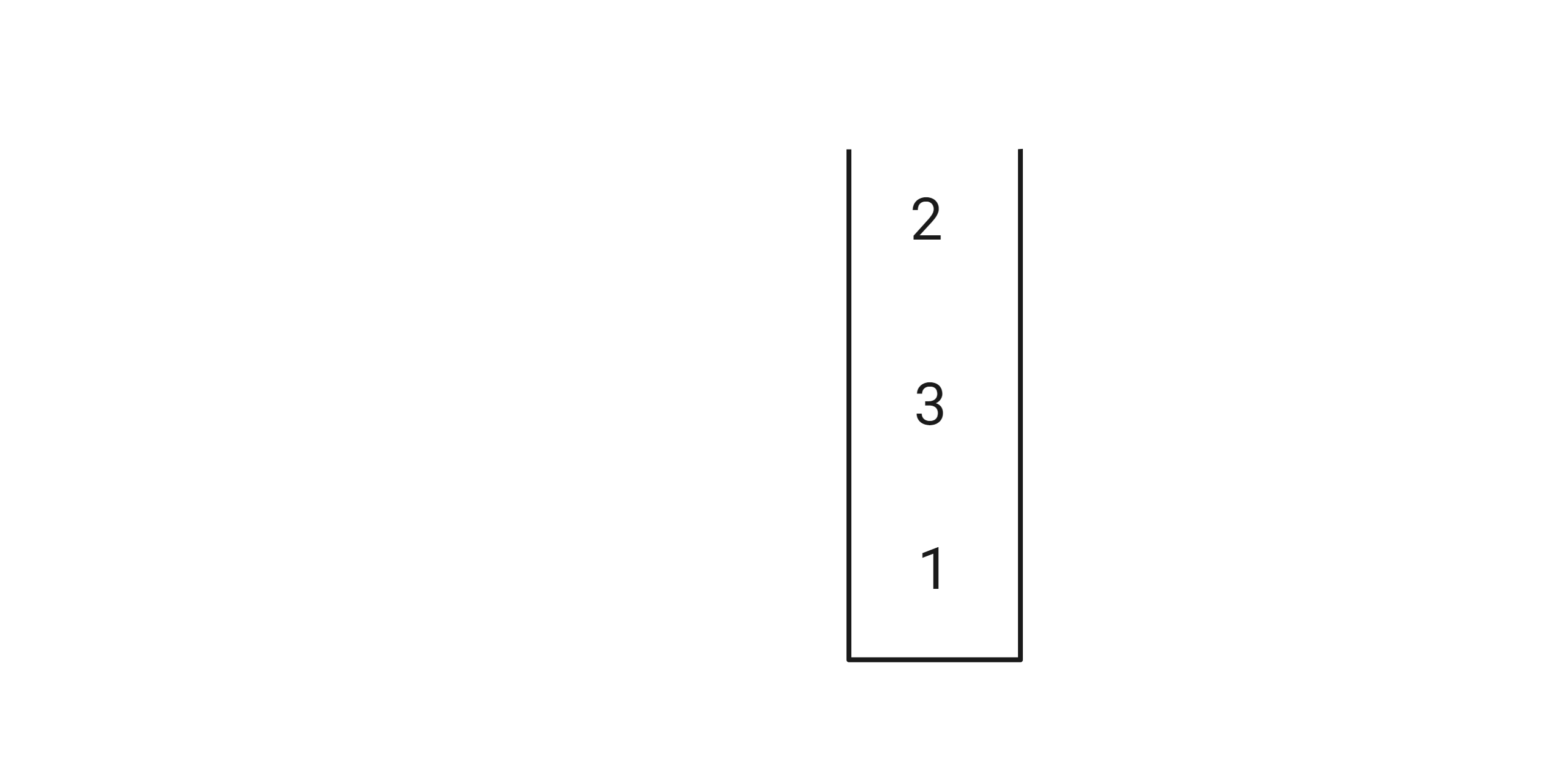0

# Sort Stack

Difficulty: EASY
Avg. time to solve
20 min
Success Rate
80%

Problem Statement
Suggest Edit

#### Note:

``````Looping through the stack is not allowed.
You need to return a stack that is sorted in descending order.
``````

#### For example,

``````Given stack S = 1 3 2
The output will be 3 2 1 since it is the sorted order.
``````

#### Input format :

``````The first line of input contains an integer ‘T’ denoting the number of test cases.
The second line of each test case contains a single integer ‘N’, denoting the stack size.
The next line contains ‘N’ space-separated integers denoting the elements of the stack, the last element of the input being the top of the stack.
If the input is 1 3 2 then the input stack will look like this :
``````#### Output format :

``````For each test case, print the stack in descending sorted order. The output of each test case will be printed in a separate line.
``````

#### Note:

``````You do not need to print anything. It has already been taken care of. Just implement the given function.
``````

#### Constraints:

``````1 <= T <= 5
1 <=  N <= 2000
0 <= S[i] <= 1000
Where ‘T’ is the total number of test cases, and 'N’ is the size of the stack and S[i] is the element of the stack.
``````

#### Sample Input 1 :

``````2
4
1 0 0 2
3
2 4 2
``````

#### Sample Output 1 :

``````2 1 0 0
4 4 2
``````

#### Explanation of the Sample Input 1:

``````For the first test case:
For the given stack, the resultant sorted stack would be 0 0 1 2.
For the second test case:
For the given stack, the resulting sorted stack would be 2 2 4.
``````

#### Sample Input 2 :

``````2
4
1 2 3 4
3
5 1 2
``````

#### Sample Output 2 :

``````4 3 2 1
5 2 1
``````Want to solve this problem? Login now to get access to solve the problems